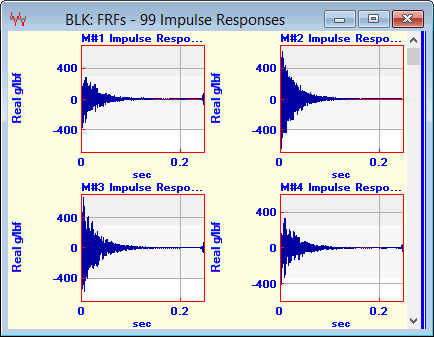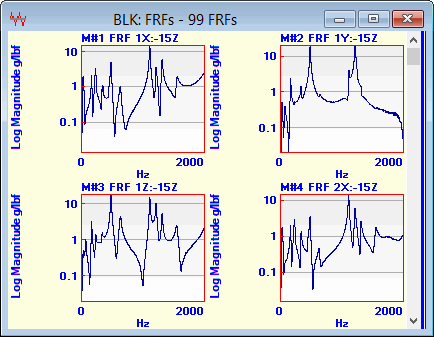# Transform | FFT

Applies the Fast Fourier Transform (FFT ) algorithm to all time domain measurements in a Data Block to transform each of them into its frequency spectrum (DFT ).

The FFT is a loss-less (also called one-to-one & onto) transformation from one domain (time or frequency) to the other
An original time waveform can always be recovered by applying the Inverse FFT to its DFT.

## Prime Number FFT

ME'scope uses a prime number FFT, which does not required that the Block Size (number of samples) be equal to a power of 2.Data Block Before FFT.Data Block After FFT.

## One Sided Versus Two Sided FFT

All of the energy in a time domain signal is spread of all frequencies, including both positive & negative frequencies in its DFT.  The FFT always calculates a Two Sided FFT, where half of a signal is represented by positive frequencies, and half by negative frequencies in its spectrum.  Therefore, a One Sided FFT yields spectrum values that are twice the values of a Two Sided FFT.  The frequency spectrum of a real valued time waveform is symmetric about zero frequency (DC), so only the positive frequency half of the spectrum is displayed.

The amplitude & power values of a DFT calculated with a Two Sided FFT are only half of the values of the its corresponding time waveform
The amplitude & power values of a DFT calculated with a One Sided FFT are the same values as its corresponding time waveform.